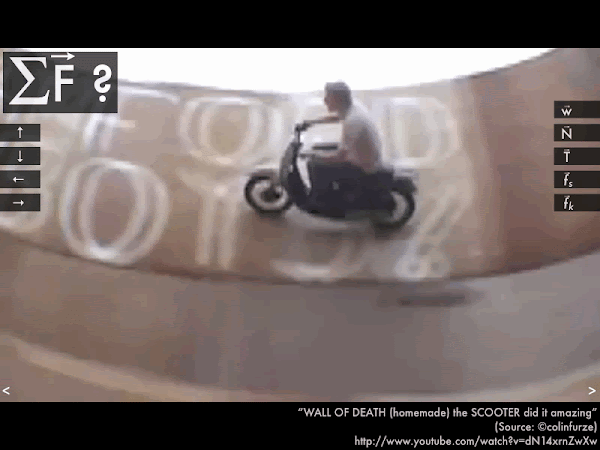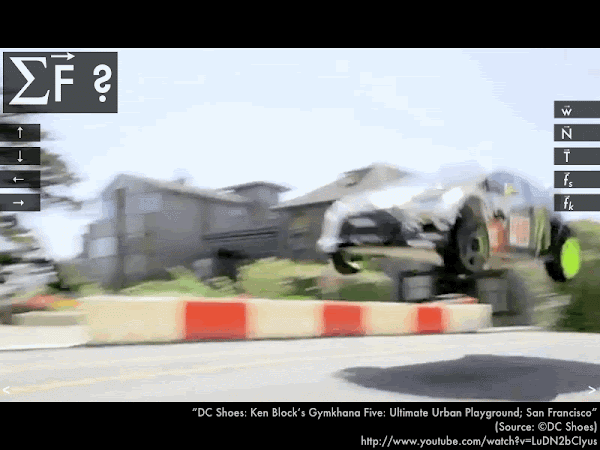## 20190918

### Online reading assignment: uniform circular motion

Physics 205A, fall semester 2019
Cuesta College, San Luis Obispo, CA

Students have a bi-weekly online reading assignment (hosted by SurveyMonkey.com), where they answer questions based on reading their textbook, material covered in previous lectures, opinion questions, and/or asking (anonymous) questions or making (anonymous) comments. Full credit is given for completing the online reading assignment before next week's lecture, regardless if whether their answers are correct/incorrect. Selected results/questions/comments are addressed by the instructor at the start of the following lecture.

The following questions were asked on reading textbook chapters and previewing a presentation on uniform circular motion.Selected/edited responses are given below.

Describe what you understand from the assigned textbook reading or presentation preview. Your description (2-3 sentences) should specifically demonstrate your level of understanding.
"In circular motion, there is a net force that produces the acceleration of an object. The centripetal force always points towards the middle of a circle."

"That circular motion is covered by Newton's second law. Even restricted to uniform circular motion Newton's second law still applies."

"I understand that by Newton's second law, there must be some type of force to create centripetal acceleration. It makes sense to me that this force is pointing towards the center of the circle, as if the force pointed outward, the object would drift off its circular path."

"Since the word 'centripetal' means 'moving toward a center,' the centripetal acceleration vector always points toward the center of the circle and continually changes direction as the object moves. For an object in uniform circular motion, the velocity vector has different directions at different places on the circle. Uniform circular motion along the arc of an infinitely large circle entails no acceleration, because it is just like motion at a constant speed along a straight line. An object undergoing uniform circular motion can never be at equilibrium because there is acceleration since the direction is constantly changing. Sometimes the centripetal force consists of a single force such as tension, friction, the normal force or a component thereof or the gravitational force."

"The concept of centripetal force, the net force of an object undergoing circular motion with a specific direction which is in towards the center of the circle and specific magnitude m·v2/r. Centripetal means 'center-seeking.' This is the requirement for uniform circular motion to maintain a constant speed along a circular trajectory with acceleration directed in towards the center."

"Centripetal force! It's like centrifugal force but not. In all seriousness, a sample scenario is a car turning a corner. This change in direction of velocity requires a net force to keep pulling the car into the center of its curve."

Describe what you found confusing from the assigned textbook reading or presentation preview. Your description (2-3 sentences) should specifically identify the concept(s) that you do not understand.
"I don't understand the conceptual understanding of why the net force points towards the center of the circle."

"I'm a little confused on if this is the same or different from 'centrifugal force.'"

"I understand the majority of these situations, but the one about the girl swinging is tripping me up."

"Slightly confused on calculating ALL the net forces during circular motion."

"Friction and how it affects centripetal force (i.e. car driving in a curve along a track)."

"How circular motion works for a car drifting around a turn. I do not understand what direction the net force is going."

"Is centripetal acceleration the acceleration the object is going at each new direction in a circular motion? I'm a little perplexed by that concept."

"I guess I still haven't grasped the idea of vertical circular motion yet."

"I'm pretty sure that I understood everything in the chapter."For the "drifting" car (skidding around a circular track at constant speed), Newton's __________ law applies to its motion, and the forces acting on it add up to a net force that:
 first; is zero. ******  second; points to the left. ***************  second; points to the right. ************************  (Unsure/lost/guessing/help!) *At the moment when the woman is at the bottom of her swinging trajectory (when the rope is vertical), Newton's __________ law applies to her motion, and the forces acting on her add up to a net force that:
 first; is zero. ******  second; points upwards. ****************************  second; points downwards. ***********  (Unsure/lost/guessing/help!) *At the moment when the motor scooter is on the left side of the screen (traveling out at you), Newton's __________ law applies to its motion, and the forces acting on it add up to a net force that:
 first; is zero. **  second; points to the left. **************  second; points to the right. **************************  (Unsure/lost/guessing/help!) ****At the moment when the car is at the very top of the loop-the-loop, Newton's __________ law applies to its motion, and the forces acting on it add up to a net force that:
 first; is zero. *****  second; points upwards. *******  second; points downwards. ********************************  (Unsure/lost/guessing/help!) **At the moment when a person is at the right edge of the screen (traveling out at you), Newton's __________ law applies to his/her motion, and the forces acting on him/her add up to a net force that:
 first; is zero. ****  second; points to the left. ****************************  second; points to the right. ***********  (Unsure/lost/guessing/help!) ***At the moment when the car is at the very top of its mid-air trajectory, Newton's __________ law applies to its motion, and the forces acting on it add up to a net force that:
 first; is zero. ********  second; points upwards. ******  second; points downwards. ****************************  (Unsure/lost/guessing/help!) ****At the moment when the skateboarder is at the very top of his mid-air trajectory, Newton's __________ law applies to his motion, and the forces acting on him add up to a net force that:
 first; is zero. ******  second; points upwards. ******  second; points downwards. *******************************  (Unsure/lost/guessing/help!) *** 

Ask the instructor an anonymous question, or make a comment. Selected questions/comments may be discussed in class.
"I feel like to really grasp what these questions are asking we need a bit more clarification in class because it's easy to get mixed up." (That's okay, just try your best--I need to see how well you do on the first try, so I know how basic or advanced to tailor my lecture for this material. (Spoiler alert: very basic.)

I've got no idea how I did on those last questions. May we go over these questions in class? I think I got all of them wrong."

"How do I know if I got these questions right or not?" (We'll go over these in class, and as part of the next reading assignment, you have a link to click on so you can see these correct answers.)

"Can we go over how to tell which way the net force is pointing when objects are moving in a circle? How do you figure out which way the net force points?" (For objects undergoing uniform circular motion (moving in a circle with constant speed), the net force always points inwards, towards the center of the circle. Always. Points. Inwards.)

"So lost help!!"

"After going over the questions I am really confused on the idea of objects in motion. Do we determine the law at a specific moment in time or the entire sequence?" (At that one moment in time. Then for an entire sequence, it's just a whole bunch of consecutive moments in time, taken one by one.)

"I am still confused about the car and motorcycle skidding around a circular track--if I was to compare the motorcycle to the car, the motorcycle would almost be in a standstill, but compared to an outside frame of reference, they both move?" (Yes, since both the car and motorcycle are accelerating (constant speed, but changing direction), they are not proper inertial reference frames, and they will not be able to properly apply Newton's laws to their motions. As an outside (stationary) observer, you would be in an inertial reference frame, and would be able to properly apply Newton's laws to them as they undergo uniform circular motion.)

"I didn't really understand how when in circular motion we are feeling the forces that may feel like outward but really they are going in." (When you undergo uniform circular motion, you are not in a proper inertial reference frame, and cannot properly apply Newton's laws because you experience "pseudoforces" like something is pulling/pushing you outwards. An outside (stationary) observer would be in an inertial reference frame, and would be able to properly apply Newton's laws and describe the net force as inwards, despite what you (undergoing uniform circular motion) "perceives.")

"Is it still considered uniform circular motion if it is not a perfect circle? What about a racetrack that is an oval in shape?" (If we can approximate part of the oval as a circle (for any small enough section of an oval), then we can apply Newton's second law for uniform circular motion for that section.)

"Do we need to memorize all the weight, normal, tension, static friction, kinetic friction, Newton's laws and ΣF = m·a equations, or are they given on quizzes and exams like the kinematic equations of motion?" (You need to memorize w = m·g, fs, max = μs·N, fk = μk·N, ΣF = m·a, and "POF-OST-ITO." Instead of just giving you those equations on a quiz or exam, I think memorizing those equations will force you understand how and why to use them while applying Newton's laws on free-body diagrams. (Note that the normal force N and the tension force T don't have any equations; their magnitudes have to be deduced, as they vary depending on the particular situation.)